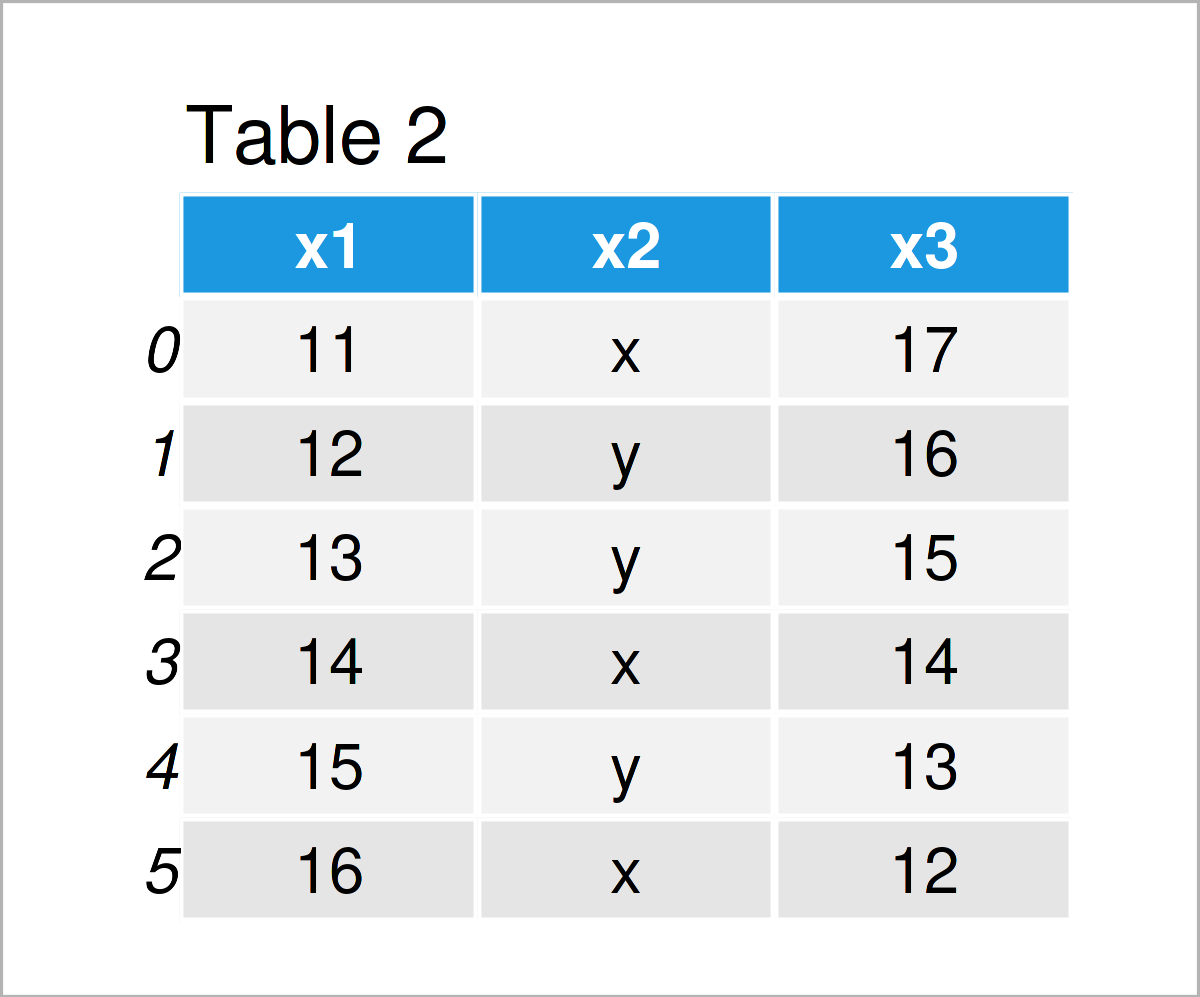# Read Multiple CSV Files & Append into One pandas DataFrame in Python (Example)

In this tutorial, I’ll explain how to import multiple CSV files and combine them into a single pandas DataFrame in Python.

The page contains these contents:

It’s time to dive into the exemplifying Python code!

## Example Data & Add-On Libraries

If we want to use the functions of the pandas library, we first have to load pandas:

`import pandas as pd                                # Load pandas`

Next, we’ll also need to construct some data that we can use in the example below:

```data1 = pd.DataFrame({'x1':range(1, 7),            # Create first pandas DataFrame
'x2':['a', 'b', 'c', 'd', 'e', 'f'],
'x3':range(7, 1, - 1)})
print(data1)                                       # Print first pandas DataFrame``````data2 = pd.DataFrame({'x1':range(11, 17),          # Create second pandas DataFrame
'x2':['x', 'y', 'y', 'x', 'y', 'x'],
'x3':range(17, 11, - 1)})
print(data2)                                       # Print second pandas DataFrame``````data3 = pd.DataFrame({'x1':range(101, 107),        # Create third pandas DataFrame
'x2':['q', 'w', 'e', 'e', 'w', 'q'],
'x3':range(107, 101, - 1)})
print(data3)                                       # Print third pandas DataFrame```As shown in Tables 1, 2, and 3, the previous Python programming syntax has constructed three pandas DataFrames. Each of these DataFrames contains the same column names, but different values.

```data1.to_csv('data1.csv', index = False)           # Export pandas DataFrames to three CSVs
data2.to_csv('data2.csv', index = False)
data3.to_csv('data3.csv', index = False)```

After we have executed the previous Python code, three new CSV files are appearing in our current working directory. These CSV files will be used as a basis for the following example.

## Example: Import Multiple CSV Files & Concatenate into One pandas DataFrame

The following Python programming syntax shows how to read multiple CSV files and merge them vertically into a single pandas DataFrame.

For this task, we first have to create a list of all CSV file names that we want to load and append to each other:

`file_names = ['data1.csv', 'data2.csv', 'data3.csv'] # Create list of CSV file names`

In the next step, we can use a for loop to read and join all our data sets into a single pandas DataFrame.

Note that I’m also using the reset_index function to reset the index numbers in our concatenated data. This is an optional step, though.

```data_all = pd.concat((pd.read_csv(i) for i in file_names)).reset_index(drop = True) # Import
print(data_all)                                    # Print combined pandas DataFrame```The output of the previous Python code is shown in Table 4 – We have created a new pandas DataFrame that contains all the rows in our three input CSV files.

## Video, Further Resources & Summary

Have a look at the following video on my YouTube channel. In the video, I’m explaining the contents of this article in a programming session.

In addition, you might want to read the other tutorials which I have published on this website.

To summarize: In this Python tutorial you have learned how to read several CSV files and combine them into a single pandas DataFrame. In case you have any additional questions, please let me know in the comments below.

Subscribe to the Statistics Globe Newsletter